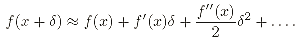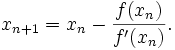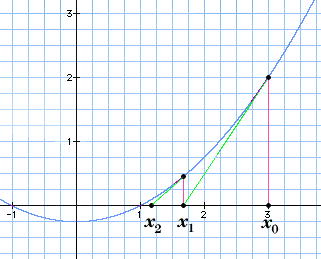# Newton method

 newton method deadline online download screenshots how to find roots numerical methods Newton C code Newton in-depth plot graphs support center

Numerical methods

Root bracketing methods

Root polishing methods

Newton method is the standard root-polishing algorithm.

Newton method, also called the Newton-Raphson method, is a root-finding algorithm that uses the first few terms of the Taylor series of a function f(x) in the vicinity of a suspected root.Newton algorithm begins with an initial guess for the location of the root. On each iteration, a line tangent to the function f is drawn at that position. The point where this line crosses the x-axis becomes the new guess.Newton method converges quadratically for single roots, and linearly for multiple roots.The function below, written in Pascal, takes a simpler aproach, ignoring the situation in which Newton method does not converge. For a C implementation of Newton method, grasp Newton.c.

PROCEDURE Newton(c, eps:Real; VAR xsol:Real);
VAR
d:Real;
BEGIN
{ df(x) = value of the first derivative }
{ eps = accuracy of the root, e.g.: 0.000001 }
REPEAT
d:=c;
c:=c-f(c)/df(c)
UNTIL Abs(d-c)<eps;
xsol:=c
END; {Newton method - Pascal code}

To find more about Newton method, visit the pages below:

See how Newton method works, solve equations free and take the results with you.

DeadLine OnLine - free equation solver. Copyright 2003-2007 Ionut Alex. Chitu. | Contact | Sitemap